## 5.11Boundary ﬂuxes

The equations in Sec. 5.10 are used to compute the velocity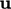, ﬂux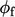and pressure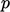based on conservation of mass and momentum. At boundaries, the ﬂux corrector Eq. (5.18 ) must calculate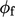in a manner consistent withandand their respective boundary conditions.

For example, at an impermeable stationary wall the calculated ﬂux must be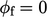, consistent with the no-slip condition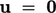. At boundary faces,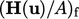and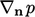must be compatible to evaluate the correctaccording to Eq. (5.18 ).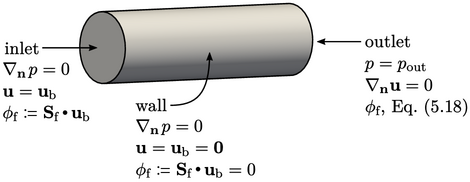The ﬁgure shows the fundamental boundary conditions from Sec. 4.3 and corresponding ﬂux evaluations. At the inlet and wall boundaries,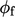is directly assigned from the boundary velocity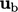by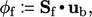within the ﬂux corrector Eq. (5.18 ).

In the absence of body forces, the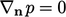boundary condition is commonly applied, as discussed in Sec. 4.4 . The ﬂux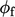is then equivalent to assigning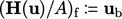in Eq. (5.18 ).

At the outlet,is not prescribed since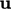is not a ﬁxed value condition. Instead,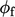is evaluated from Eq. (5.18 ) using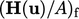taken from cells adjacent to the boundary and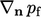calculated on the boundary.

### Fluxes with a body force

When a body force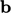is present in the momentum equation, the gradient condition for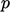, e.g. at an inlet or wall, in principle becomes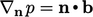, as discussed in Sec. 4.4 . The precise details of the boundary condition in fact depend on howis incorporated within the coupling algorithm, discussed below.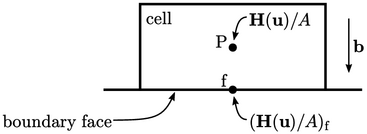One approach, illustrated by the algorithm in Sec. 5.10 , is to include the body force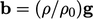within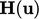in Eq. (5.16 ). In that case, the assignment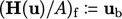cannot be valid, so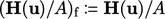is adopted instead.

With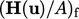established, the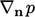condition is calculated based on the known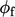by inverting Eq. (5.18 ). This approach causes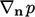to include a contribution from viscous stresses, as in Eq. (4.5 ), which may cause instability.

To avoid this problem,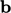is omitted from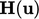in Eq. (5.16 ), appearing instead as an extra term in the other equations in Sec. 5.10 , e.g. the ﬂux corrector Eq. (5.18 ) which becomes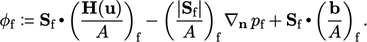(5.20)
Assigning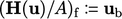at boundaries where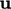is ﬁxed satisﬁes Eq. (5.20 ) when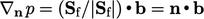. This is the condition ignoring viscous stresses described in Sec. 4.4 .
Notes on CFD: General Principles - 5.11 Boundary ﬂuxes